# SPARSE-MOD COVID-19 Model

#### July 2022

library(SPARSEMODr)
library(future.apply)
library(tidyverse)
library(viridis)
library(lubridate)

# To run in parallel, use, e.g., plan("multisession"):
future::plan("sequential")

## The COVID-19 Example Model

Here we present a walk-through of using the SPARSE-MOD COVID-19 Model, which represents simplified dynamics of transmission of SARS-CoV-2 and COVID-19 progression of patients through the hospital system. The model does not include multiple viral variants, nor does it include vaccination. Therefore, we personally recommend using this R package in an exploratory and educational capacity, not as a mechanism with which to forecast or project disease dynamics. See our vignette on key features of SPARSEMODr for more general details of the SPARSEMODr package. And see the end of this vignette for model equations.

### Generating a synthetic meta-population

First, we will simulate data that describes the meta-population1 of interest.

# Set seed for reproducibility
set.seed(5)

# Number of focal populations:
n_pop = 100

# Population sizes + areas
## Draw from neg binom:
census_area = rnbinom(n_pop, mu = 50, size = 3)

# Identification variable for later
pop_ID = c(1:n_pop)

# Assign coordinates, plot for reference
lat_temp = runif(n_pop, 32, 37)
long_temp = runif(n_pop, -114, -109)

# Storage:
region = rep(NA, n_pop)
pop_N = rep(NA, n_pop)

# Assign region ID and population size
for(i in 1 : n_pop){
if ((lat_temp[i] >= 34.5) & (long_temp[i] <= -111.5)){
region[i] = "1"
pop_N[i] = rnbinom(1, mu = 50000, size = 2)
} else if((lat_temp[i] >= 34.5) & (long_temp[i] > -111.5)){
region[i] = "2"
pop_N[i] = rnbinom(1, mu = 10000, size = 3)
} else if((lat_temp[i] < 34.5) & (long_temp[i] > -111.5)){
region[i] = "4"
pop_N[i] = rnbinom(1, mu = 50000, size = 2)
} else if((lat_temp[i] < 34.5) & (long_temp[i] <= -111.5)){
region[i] = "3"
pop_N[i] = rnbinom(1, mu = 10000, size = 3)
}
}

pop_local_df =
data.frame(pop_ID = pop_ID,
pop_N = pop_N,
census_area,
lat = lat_temp,
long = long_temp,
region = region)

# Plot the map:
pop_plot = ggplot(pop_local_df) +
geom_point(aes(x = long, y = lat,
fill = region, size = pop_N),
shape = 21) +
scale_size(name = "Pop. Size", range = c(1,5),
breaks = c(5000, 50000, 150000)) +
scale_fill_manual(name = "Region", values = c("#00AFBB", "#D16103",
"#E69F00", "#4E84C4")) +
geom_hline(yintercept = 34.5, colour = "#999999", linetype = 2) +
geom_vline(xintercept = -111.5, colour = "#999999", linetype = 2) +
guides(size = guide_legend(order = 2),
fill = guide_legend(order = 1,
override.aes = list(size = 3))) +
# Map coord
coord_quickmap() +
theme_classic() +
theme(
axis.line = element_blank(),
axis.title = element_blank(),
plot.margin = unit(c(0, 0.1, 0, 0), "cm")
)

pop_plot

# Calculate pairwise dist
## in meters so divide by 1000 for km
dist_mat = geosphere::distm(cbind(pop_local_df$long, pop_local_df$lat))/1000
hist(dist_mat, xlab = "Distance (km)", main = "")

# We need to determine how many Exposed individuals
# are present at the start in each population
E_pops = vector("numeric", length = n_pop)
# We'll assume a total number of exposed across the
# full meta-community, and then randomly distribute these hosts
n_initial_E = 20
# (more exposed in larger populations)
these_E <- sample.int(n_pop,
size = n_initial_E,
replace = TRUE,
prob = pop_N)
for(i in 1:n_initial_E){
E_pops[these_E[i]] <- E_pops[these_E[i]] + 1
}

pop_local_df$E_pops = E_pops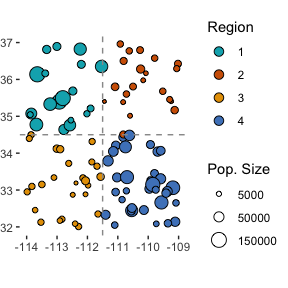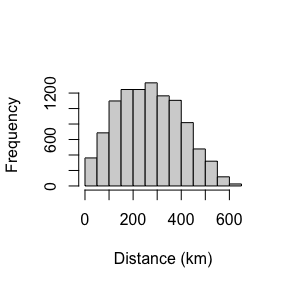### Setting up the time-windows One of the benefits of the SPARSEMODr is that the user can specify how the values of certain parameters of the model change over time (see our vignette on key features of SPARSEMODr for more details). We demonstrate this below, where we allow the time-varying transmission rate, $$\beta_{t}$$, to change in a step-wise fashion due to, for instance, changes in the behavior of the host population. In this case, we assume the transmission rate changes during discrete blocks of time, or time windows. When the parameter values change between two time windows, the model imputes a linear change over the number of days in that window. In other words, the user specifies the value of the parameter achieved on the last day of the time window. In other vignettes, we show how the user can instead specify daily values of the time-varying parameters, which allows for more flexibility. Here, we also assume host migration dynamics are invariable across time. We’ll use the $$\texttt{time_windows()}$$ function to generate a pattern of $$\beta_{t}$$ that looks like the following: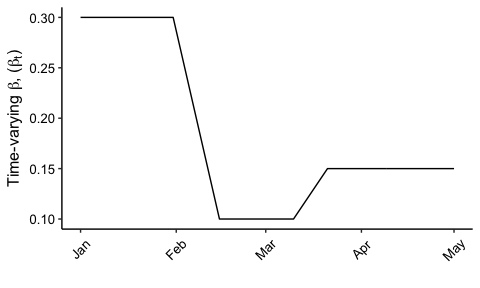Importantly, SPARSEMODr allows the user to assign unique patterns of time-varying $$\beta$$ for each population. Below, we will assume that the pattern of $$\beta_{t}$$ is unique for each region on the map, above. Correspondingly, each population within the region of interest will have the same pattern of $$\beta_{t}$$. In this scenario, then, each region has different transmission dynamics, and movement of hosts among regions can influence the local (i.e., within a single population) and regional patterns of disease. # Set up the dates of change. 5 time windows n_windows = 5 ## Specify the start and end dates of the time intervals start_dates = c(mdy("1-1-20"), mdy("2-1-20"), mdy("2-16-20"), mdy("3-11-20"), mdy("3-22-20")) end_dates = c(mdy("1-31-20"), mdy("2-15-20"), mdy("3-10-20"), mdy("3-21-20"), mdy("5-1-20")) ### TIME-VARYING PARAMETERS ### # beta pattern per region region_beta = list( "1"=c(0.30, 0.10, 0.10, 0.15, 0.15), "2"=c(0.30, 0.08, 0.08, 0.10, 0.10), "3"=c(0.30, 0.12, 0.12, 0.19, 0.19), "4"=c(0.30, 0.03, 0.03, 0.12, 0.12) ) ## Assign the appropriate, regional pattern of beta ## to each population changing_beta = vector("list", length = n_pop) for (this_pop in 1:n_pop) { this_region <- pop_local_df$region[this_pop]
changing_beta[[this_pop]] <- region_beta[[this_region]]
}

# Migration rate
changing_m = rep(1/10.0, times = n_windows)
# Migration range
changing_dist_phi = rep(150, times = n_windows)
# Immigration (none)
changing_imm_frac = rep(0, times = n_windows)

# Create the time_window() object
tw = time_windows(
beta = changing_beta,
m = changing_m,
dist_phi = changing_dist_phi,
imm_frac = changing_imm_frac,

start_dates = start_dates,
end_dates = end_dates
)

# Create the covid19_control() object
covid19_control <- covid19_control(input_N_pops = pop_N,
input_E_pops = E_pops)
#> Parameter input_I_asym_pops was not specified; assuming to be zeroes.
#> Parameter input_I_presym_pops was not specified; assuming to be zeroes.
#> Parameter input_I_sym_pops was not specified; assuming to be zeroes.
#> Parameter input_I_home_pops was not specified; assuming to be zeroes.
#> Parameter input_I_hosp_pops was not specified; assuming to be zeroes.
#> Parameter input_I_icu1_pops was not specified; assuming to be zeroes.
#> Parameter input_I_icu2_pops was not specified; assuming to be zeroes.
#> Parameter input_R_pops was not specified; assuming to be zeroes.
#> Parameter input_D_pops was not specified; assuming to be zeroes.

# Date Sequence for later:
date_seq = seq.Date(start_dates, end_dates[n_windows], by = "1 day")

### Running the COVID-19 model in parallel

Now we have all of the input elements needed to run SPARSEMODr’s COVID-19 model. Below we demonstrate a workflow to generate stochastic realizations of the model in parallel.

# How many realizations of the model?
n_realz = 75

# Need to assign a distinct seed for each realization
## Allows for reproducibility
input_realz_seeds = c(1:n_realz)

# Run the model in parallel

model_output =
model_parallel(
# Necessary inputs
input_dist_mat = dist_mat,
input_census_area = pop_local_df$census_area, input_tw = tw, input_realz_seeds = input_realz_seeds, control = covid19_control, # OTHER MODEL PARAMS trans_type = 1, # freq-dependent trans stoch_sd = 2.0 # stoch transmission sd ) glimpse(model_output) #> Rows: 915,000 #> Columns: 19 #>$ pops.seed         <int> 1, 1, 1, 1, 1, 1, 1, 1, 1, 1, 1, 1, 1, 1, 1, 1, 1, 1…
#> $pops.pop <int> 1, 2, 3, 4, 5, 6, 7, 8, 9, 10, 11, 12, 13, 14, 15, 1… #>$ pops.time         <int> 1, 1, 1, 1, 1, 1, 1, 1, 1, 1, 1, 1, 1, 1, 1, 1, 1, 1…
#> $pops.S_pop <int> 118910, 8765, 10066, 86540, 11709, 14078, 10111, 538… #>$ pops.E_pop        <int> 1, 0, 0, 2, 1, 0, 0, 0, 0, 0, 0, 0, 1, 1, 0, 0, 0, 0…
#> $pops.I_asym_pop <int> 0, 0, 0, 0, 0, 0, 0, 0, 0, 0, 0, 0, 0, 0, 0, 0, 0, 0… #>$ pops.I_presym_pop <int> 0, 0, 0, 0, 0, 0, 0, 0, 0, 0, 0, 0, 0, 0, 0, 0, 0, 0…
#> $pops.I_sym_pop <int> 0, 0, 0, 0, 0, 0, 0, 0, 0, 0, 0, 0, 0, 0, 0, 0, 0, 0… #>$ pops.I_home_pop   <int> 0, 0, 0, 0, 0, 0, 0, 0, 0, 0, 0, 0, 0, 0, 0, 0, 0, 0…
#> $pops.I_hosp_pop <int> 0, 0, 0, 0, 0, 0, 0, 0, 0, 0, 0, 0, 0, 0, 0, 0, 0, 0… #>$ pops.I_icu1_pop   <int> 0, 0, 0, 0, 0, 0, 0, 0, 0, 0, 0, 0, 0, 0, 0, 0, 0, 0…
#> $pops.I_icu2_pop <int> 0, 0, 0, 0, 0, 0, 0, 0, 0, 0, 0, 0, 0, 0, 0, 0, 0, 0… #>$ pops.R_pop        <int> 0, 0, 0, 0, 0, 0, 0, 0, 0, 0, 0, 0, 0, 0, 0, 0, 0, 0…
#> $pops.D_pop <int> 0, 0, 0, 0, 0, 0, 0, 0, 0, 0, 0, 0, 0, 0, 0, 0, 0, 0… #>$ events.pos        <int> 1, 0, 0, 1, 0, 0, 0, 0, 0, 0, 0, 0, 0, 0, 0, 0, 0, 0…
#> $events.sym <int> 0, 0, 0, 0, 0, 0, 0, 0, 0, 0, 0, 0, 0, 0, 0, 0, 0, 0… #>$ events.total_hosp <int> 0, 0, 0, 0, 0, 0, 0, 0, 0, 0, 0, 0, 0, 0, 0, 0, 0, 0…
#> $events.total_icu <int> 0, 0, 0, 0, 0, 0, 0, 0, 0, 0, 0, 0, 0, 0, 0, 0, 0, 0… #>$ events.n_death    <int> 0, 0, 0, 0, 0, 0, 0, 0, 0, 0, 0, 0, 0, 0, 0, 0, 0, 0…

### Plotting the output

First we need to manipulate and aggregate the output data. Here we show an example just using the ‘new events’ that occur each day.

# Grab the new events variables
new_events_df =
model_output %>%
select(pops.seed:pops.time, events.pos:events.n_death)

# Simplify/clarify colnames
colnames(new_events_df) = c("iter","pop_ID","time",
"new_pos", "new_sym", "new_hosp",
"new_icu", "new_death")
# Join the region
region_df = pop_local_df %>% select(pop_ID, region)
new_events_df =
left_join(new_events_df, region_df, by = "pop_ID")

# Join with dates (instead of "time" integer)
date_df = data.frame(
date = date_seq,
time = c(1:length(date_seq))
)
new_events_df =
left_join(new_events_df, date_df, by = "time")

# Aggregate outcomes by region:
## First, get the sum across regions,dates,iterations
new_event_sum_df =
new_events_df %>%
group_by(region, iter, date) %>%
summarize(new_pos = sum(new_pos),
new_sym = sum(new_sym),
new_hosp = sum(new_hosp),
new_icu = sum(new_icu),
new_death = sum(new_death))
#> summarise() has grouped output by 'region', 'iter'. You can override using the .groups argument.
glimpse(new_event_sum_df)
#> Rows: 36,600
#> Columns: 8
#> Groups: region, iter 
#> $region <chr> "1", "1", "1", "1", "1", "1", "1", "1", "1", "1", "1", "1", … #>$ iter      <int> 1, 1, 1, 1, 1, 1, 1, 1, 1, 1, 1, 1, 1, 1, 1, 1, 1, 1, 1, 1, …
#> $date <date> 2020-01-01, 2020-01-02, 2020-01-03, 2020-01-04, 2020-01-05,… #>$ new_pos   <int> 0, 1, 3, 0, 0, 0, 0, 1, 0, 2, 1, 5, 1, 1, 3, 2, 14, 5, 6, 2,…
#> $new_sym <int> 0, 0, 0, 1, 0, 0, 0, 0, 1, 1, 1, 1, 2, 3, 1, 2, 1, 4, 4, 5, … #>$ new_hosp  <int> 0, 0, 0, 0, 0, 0, 0, 0, 0, 0, 0, 0, 0, 0, 0, 0, 0, 1, 0, 1, …
#> $new_icu <int> 0, 0, 0, 0, 0, 0, 0, 0, 0, 0, 0, 0, 0, 0, 0, 0, 0, 0, 0, 0, … #>$ new_death <int> 0, 0, 0, 0, 0, 0, 0, 0, 0, 0, 0, 0, 0, 0, 0, 0, 0, 0, 0, 0, …

# Now calculate the median model trajectory across the realizations
new_event_median_df =
new_event_sum_df %>%
ungroup() %>%
group_by(region, date) %>%
summarize(med_new_pos = median(new_pos),
med_new_sym = median(new_sym),
med_new_hosp = median(new_hosp),
med_new_icu = median(new_icu),
med_new_death = median(new_death))
#> summarise() has grouped output by 'region'. You can override using the .groups argument.
glimpse(new_event_median_df)
#> Rows: 488
#> Columns: 7
#> Groups: region 
#> $region <chr> "1", "1", "1", "1", "1", "1", "1", "1", "1", "1", "1", "… #>$ date          <date> 2020-01-01, 2020-01-02, 2020-01-03, 2020-01-04, 2020-01…
#> $med_new_pos <int> 1, 1, 1, 1, 1, 1, 1, 1, 2, 2, 3, 3, 4, 5, 5, 5, 7, 7, 9,… #>$ med_new_sym   <int> 0, 0, 0, 0, 0, 0, 1, 1, 0, 1, 1, 2, 2, 2, 2, 2, 3, 3, 4,…
#> $med_new_hosp <int> 0, 0, 0, 0, 0, 0, 0, 0, 0, 0, 0, 0, 0, 0, 0, 0, 0, 0, 0,… #>$ med_new_icu   <int> 0, 0, 0, 0, 0, 0, 0, 0, 0, 0, 0, 0, 0, 0, 0, 0, 0, 0, 0,…
#> $med_new_death <int> 0, 0, 0, 0, 0, 0, 0, 0, 0, 0, 0, 0, 0, 0, 0, 0, 0, 0, 0,… Now we’ll start creating a rather complex figure to show the different time intervals. We’ll layer on the elements. For this example, we’ll just look at the number of new hospitalizations per region. # SET UP SOME THEMATIC ELEMENTS: ## Maximum value of the stoch trajectories, for y axis ranges max_hosp = max(new_event_sum_df$new_hosp)
## Breaks for dates:
date_breaks = seq(range(date_seq),
range(date_seq),
by = "1 month")

#######################
# PLOT
#######################

# First we'll create an element list for plotting:
plot_new_hosp_base =
list(
# Date Range:
scale_x_date(limits = range(date_seq),
breaks = date_breaks, date_labels = "%b"),
# New Hosp Range:
scale_y_continuous(limits = c(0, max_hosp*1.05)),
# BOXES AND TEXT TO LABEL TIME WINDOWS
annotate("rect", xmin = start_dates, xmax = end_dates,
ymin = 0, ymax = max_hosp*1.05,
fill = "gray", alpha = 0.2),
annotate("rect", xmin = start_dates, xmax = end_dates,
ymin = 0, ymax = max_hosp*1.05,
fill = "gray", alpha = 0.2),
annotate("rect", xmin = start_dates, xmax = end_dates,
ymin = 0, ymax = max_hosp*1.05,
fill = "gray", alpha = 0.2),
# THEME ELEMENTS
labs(x = "", y = "New Hospitalizations Per Day"),
theme_classic(),
theme(
axis.text = element_text(size = 12, color = "black"),
axis.title = element_text(size = 14, color = "black"),
axis.text.x = element_text(angle = 45, vjust = 0.5)
)
)

ggplot() + plot_new_hosp_base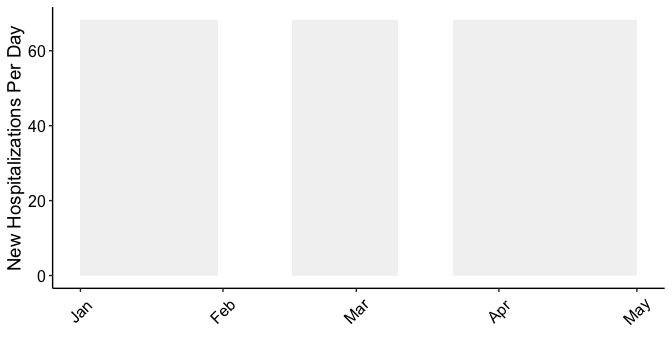Ok, now we have our plotting base, and we’ll layer on the model output. We’ll add the stochastic trajectories as well as the median model trajectory.


# region labels for facets:
region_labs = paste0("Region ",
sort(unique(region_df$region))) names(region_labs) = sort(unique(region_df$region))

# Regional beta labels
region_beta_df = data.frame(
beta_lab = paste0("beta = ",format(unlist(lapply(region_beta, function(x){x[c(1,3,5)]})),nsmall = 1)),
region = as.character(rep(c(1:4), each=3)),
date = rep(start_dates[c(1,3,5)],times=4),
new_hosp = max_hosp*1.05
)

# Create the plot:
plot_new_hosp =
ggplot() +
# Facet by Region
facet_wrap(~region,
scales = "free",
labeller = labeller(region = region_labs)) +
# Add our base thematics
plot_new_hosp_base +
# Add the stoch trajectories:
geom_path(data = new_event_sum_df,
aes(x = date, y = new_hosp, group = iter, color = region),
alpha = 0.05) +
# Add the median trajectory:
geom_path(data = new_event_median_df,
aes(x = date, y = med_new_hosp, color = region),
size = 2) +
# Add the beta labels:
geom_text(data = region_beta_df,
aes(x = date, y = new_hosp, label = beta_lab),
color = "#39558CFF", hjust = 0, vjust = 1, size = 3.0) +
# Colors per region:
scale_color_manual(values = c("#00AFBB", "#D16103",
"#E69F00", "#4E84C4")) +
guides(color="none")

plot_new_hosp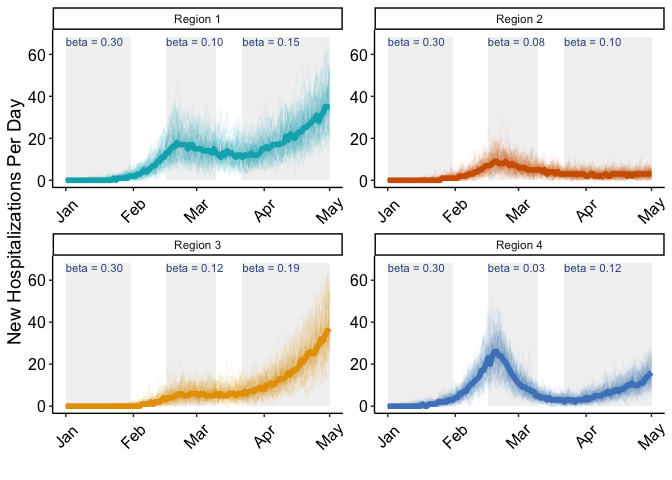## Model equations

The version of the model with frequency-dependent transmission that is implemented in each population is below. Note that there is also simulated movement dynamics of the $$S$$, $$I_a$$, $$I_p$$ and $$I_s$$ classes, moderated by a rate parameter $$m$$. However, these dynamics are not explicitly represented in these equations. \begin{align} \frac{dS}{dt} &= -\beta_{t} \lambda_{t} S \\ \frac{dE}{dt} &= \beta_{t} \lambda_{t} S - \delta_{1} E \\ \frac{dI_a}{dt} &= \delta_1 \rho_1 E - \gamma_{a} I_a \\ \frac{dI_p}{dt} &= \delta_1 (1 - \rho_1) E - \delta_2 I_p \\ \frac{dI_s}{dt} &= \delta_2 I_p - \delta_3 I_s \\ \frac{dI_b}{dt} &= \delta_3 (1 - \rho_2 - \rho_3) I_s - \gamma_{b} I_b \\ \frac{dI_h}{dt} &= \delta_3 \rho_2 I_s - \delta_4 I_h \\ \frac{dI_{c1}}{dt} &= \delta_3 \rho_3 I_s + \delta_4 \rho_4 I_h - \delta_5 I_{c1} \\ \frac{dI_{c2}}{dt} &= \delta_5 (1 - \rho_5) I_{c1} - \gamma_{c} I_{c2} \\ \frac{dD}{dt} &= \delta_5 \rho_5 I_{c1} \\ \frac{dR}{dt} &= \gamma_{a} I_a + \gamma_{b} I_b + \gamma_{c} I_{c2} + \delta_4 (1 - \rho_4) I_h \end{align}

And the time-varying force of infection: $\lambda_{t} = \frac{\omega_{1} I_a + I_p + I_s + I_b + \omega_2 \left( I_h + I_{c1} + I_{c2} \right)}{N - D}$

State Variable Description
$$S$$ Number of susceptible individuals
$$E$$ Number of exposed individuals
$$I_a$$ Number of asymptomatic individuals
$$I_p$$ Number of pre-symptomatic individuals
$$I_s$$ Number of mildly symptomatic individuals
$$I_b$$ Number of mildly symptomatic individuals on bed rest at home
$$I_h$$ Number of hospitalized individuals
$$I_{c1}$$ Number of individuals in the ICU
$$I_{c2}$$ Number of individuals in the recovery (step-down) ICU
$$D$$ Number of deceased individuals
$$R$$ Number of susceptible individuals
Parameter Description Corresponding model input
$$\beta_t$$ Time-varying transmission rate $$\texttt{beta}$$
$$\omega_1$$ Proportion reduction in transmission for asymptomatic folks $$\texttt{frac_beta_asym}$$
$$\omega_2$$ Proportion reduction in transmission for hospitalized folks $$\texttt{frac_beta_hosp}$$
$$N$$ Total number of individuals in population $$\texttt{input_N_pops}$$
$$\delta_1$$ Transition rate: exposed to pre-symptomatic $$\texttt{delta}$$
$$\delta_2$$ Transition rate: pre-symptomatic to symptomatic $$\texttt{recov_p}$$
$$\delta_3$$ Transition rate: symptomatic to home or regular hospital bed or ICU $$\texttt{recov_s}$$
$$\delta_4$$ Transition rate: regular hospital bed to home or ICU $$\texttt{recov_hosp}$$
$$\delta_5$$ Transition rate: ICU to step-down ICU $$\texttt{recov_icu1}$$
$$\gamma_{a}$$ Recovery rate: asymptomatic $$\texttt{recov_a}$$
$$\gamma_{b}$$ Recovery rate: home bed $$\texttt{recov_home}$$
$$\gamma_{c}$$ Recovery rate: step-down ICU $$\texttt{recov_icu2}$$
$$\rho_1$$ Fraction of exposed that transition to asymptomatic $$\texttt{asym_rate}$$
$$\rho_2$$ Fraction of symptomatic that transition to hospital bed $$\texttt{hosp_rate}$$
$$\rho_3$$ Fraction of symptomatic that transition to icu bed $$\texttt{sym_to_icu_rate}$$
$$\rho_4$$ Fraction of hospitalized that transition to ICU $$\texttt{icu_rate}$$
$$\rho_5$$ Fraction of patients in ICU that die of disease $$\texttt{death_rate}$$

1. A set of distinct, focal populations that are connected by migration↩︎